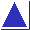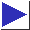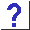# comp.lang.c FAQ list ·Question 9.2

Q: Isn't #defining TRUE to be 1 dangerous, since any nonzero value is considered ``true'' in C? What if a built-in logical or relational operator ``returns'' something other than 1?

A: It is true (sic) that any nonzero value is considered true in C, but this applies only ``on input'', i.e. where a Boolean value is expected. When a Boolean value is generated by a built-in operator such as ==, !=, and <=, it is guaranteed to be 1 or 0. Therefore, the test

```	if((a == b) == TRUE)
```
would work as expected (as long as TRUE is 1), but it is obviously silly. In fact, explicit tests against TRUE and FALSE are generally inappropriate. In particular, and unlike the built-in operators, some library functions (notably isupper, isalpha, etc.) return, on success, a nonzero value which is not necessarily 1, so comparing their return values against a single value such as TRUE is quite risky and likely not to work.

(Besides, if you believe that

```if((a == b) == TRUE)
```
is an improvement over
```if(a == b)
```
why stop there? Why not use:
```if(((a == b) == TRUE) == TRUE)
```
or even:
```if((((a == b) == TRUE) == TRUE) == TRUE)
```

Given that

```	if(a == b)
```
is a perfectly legitimate conditional, so is
```	#include <ctype.h>

...

if(isupper(c))
{ ... }
```
since isupper is known to return zero/nonzero for false/true. Similarly, there should not be any reluctance to use code like
```	int is_vegetable;		/* really a bool */

...

if(is_vegetable)
{ ... }
```
or
```	extern int fileexists(char *);	/* returns true/false */

...

if(fileexists(outfile))
{ ... }
```
as long as is_vegetable and fileexists() are of ``conceptual Boolean type.'' Alternatives like
```	if(is_vegetable == TRUE)
or
if(fileexists(outfile) == YES)
```
are not really any improvement. (They can be thought of as ``safer'' or ``better style,'' but they can also be thought of as risky or poor style. They certainly don't read as smoothly. See question 17.10.)

A good rule of thumb is to use TRUE and FALSE (or the like) only for assignment to a Boolean variable or function parameter, or as the return value from a Boolean function, but never in a comparison.